# Issue 03 launch quiz

How many points could you have scored at our launch quiz?Last night, we hosted a maths themed pub quiz at the Print Room Cafe in UCL to celebrate the launch of issue 03. If you couldn’t make it along, why not have a go at the questions now! Afterwards, you can find the answers here.

## Round zero: The picture round

#### Definitions

What does each of these define?

1. $\forall x\in A\ \ \exists\varepsilon\ \ \text{s.t.}\ \ B_\varepsilon(x)\subseteq A$
2. $\forall\varepsilon\,\exists \delta\text{ s.t. }|x-y| \lt \delta\implies|f(x)-f(y)| \lt \varepsilon$
3. $p$ is not zero or a unit and $p|ab\implies p|a$ or $p|b$
4. $\forall\varepsilon\ \ \exists N\ \ \text{s.t.}\ \ \forall n>N\ \ |x_n-L|\lt\varepsilon$

#### Maths Departments

At which university is each of these departments?1234

#### Mathematicians on money

Who are these mathematicians?12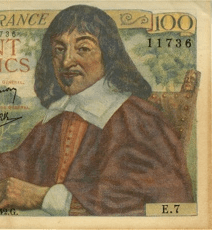34

#### Shapes

Here are the properties of four quadrilaterals ABCD (corners labelled clockwise). What
shape is each one?

1. AB and CD are parallel. BC and DA are not parallel.
2. AB = CD. Angles ABC and BCD are right angles.
3. AC and DB meet at right angles. AB ≠ CD.
4. Angles ABC and CDA are equal. Angles BCD and DAB are equal. Angle ABC is not a right angle.

## Round one: In the news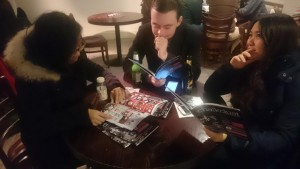Reading issue 03 before the quiz begins

The first round is all about recent mathematical news stories.

1. In November, a professor claimed to have proven the Riemann Hypothesis. Which country was he from?
2. In January, a new largest known prime number was discovered. To the nearest million, how many (decimal) digits long it is?
3. In February, it was announced that gravitational waves had been detected. The collision of two of what caused the wave which was detected?
4. In 2014, four people won Fields Medals. Name them. One…
5. …point…
6. …for…
7. each.
8. Whose sweet’s went viral after a difficult GCSE maths question last year?
9. What was Pietro Boselli in the news for last year?
10. In December, a paper was published revealing a new way to slice what into equal pieces?

## Round two: Two-for-one round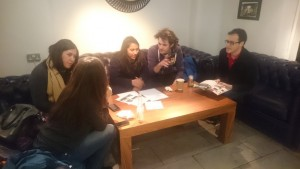In this round there are 12 questions, but only 6 answers. This is because each answer appears twice. This is a format borrowed from the excellent Two-for-one quiz at The Oxford, Kentish Town.

1. Who is Chalkdust magazine’s agony uncle?
2. Which mathematician used the pseudonym Monsieur Antoine-August Le Blanc to study at Ecole Polytchnique, where women were not at the time allowed to study?
3. What is the multiplicative identity in the ring of integers?
4. What is the additive identity in the ring of integers?
5. A certain type of boundary condition prescribes the value of the function’s derivative on the boundary. After which mathematician are these boundary conditions named?
6. Another type of boundary condition prescribes the value of the function on the boundary. After which mathematician are these boundary conditions named?
7. After which mathematician are numbers of the form 4n+1 named?
8. Which mathematician published a list of 23 important unsolved problems in 1900?
9. In 2000, a list of seven Millennium Prize Problems was published. How many of these have been solved?
10. Alfred Clebsch and which other mathematician founded the mathematical journal Mathematische Annalen?
11. After which mathematician are primes, p, such that 2p+1 is also prime named?
12. What is -459.67 degrees Fahrenheit in degrees Kelvin?

## Round three: The wipeout round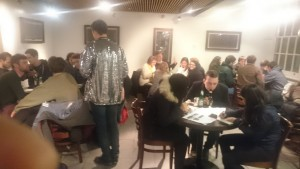A view of the quizmaster’s excellent sparkly jacket.

In this round, you are given the names of 20 mathematical papers. 10 of them are real papers. 10 of them are completely made up. You get three points for each correct one you spot, but if you write down any incorrect answers, you get no points for the whole round!

• On the coupling of boundary integral and finite element methods
• The fluid dynamics of the chocolate fountain
• Pseudo-inverses of non-square matrices
• The chocolate dynamics of the fluid fountain
• Modular elliptic curves and Fermat’s Last Theorem
• Nineteen dubious ways to compute the exponential of a matrix
• Isomorphic elliptic manifolds
• Nine dubious ways to compute the exponential of a matrix
• Cutting down trees with a Markov chainsaw
• On the application of geometry to the creation of jokes
• A mathematical theory of conversation
• When zombies attack!: Mathematical modelling of an outbreak of zombie infection
• Generalized Calabi-Yau manifolds
• The modelling of the downhill cyclist with fluid dynamics
• A mathematical theory of communication
• Galois groupies, diameters and chords: What rock and roll can learn from mathematics
• Ring theoretic properties of certain Hecke algebras
• Quasi-steady-state approximates for chemical reaction networks
• Galois cohomologies of p-adic Lie groups
• Mathematical modelling of soap bubble diffusion

## Round four: Numbers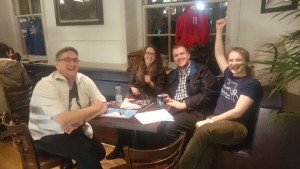The winning team.

In this round, each answer is a number. To give you a little bit of help, the answers appear in ascending order.

1. How many bits are there in a byte?
2. What is the atomic number of sulphur?
3. What is the smallest odd prime which is not a twin prime?
4. What is the answer to the ultimate question of life the universe and everything?
5. What is the highest numbered ball in the UK Lotto?
6. In cricket, which score is known as a ‘Nelson’?
7. How many hours are there in the title of a 2010 Danny Boyle film?
8. How many yards are there in a furlong?
9. In what year was the great fire of London
10. GH Hardy famously told Srinivasa Ramanujan that the number of his taxi had been uninteresting. Ramanujan replied that the number was in the smallest number that can be written as the sum of two cubes in two ways. What was the number?

Now that you’ve finished, you can check your answers here. If you enjoyed these, why not have a go at our fiendish issue 03 prize crossnumber.Matthew is a postdoctoral researcher at University College London. He hasn’t had time to play Klax since the noughties, but he’s pretty sure that Coke is it!